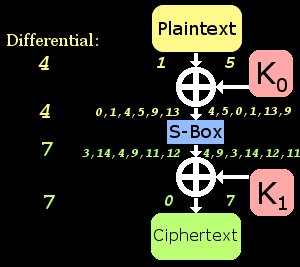# 差分密码分析简介

written by oneman233
2022-10-25

//Jon King
//Differential Cryptanalysis Toy Implementation

#include <stdio.h>

int sbox = {3, 14, 1, 10, 4, 9, 5, 6, 8, 11, 15, 2, 13, 12, 0, 7};
int sboxRev = {14, 2, 11, 0, 4, 6, 7, 15, 8, 5, 3, 9, 13, 12, 1, 10};
int chars;

int roundFunc(int input, int key)
{
return sbox[key ^ input];
}

int encrypt(int input, int k0, int k1)
{
int x0 = roundFunc(input, k0);
return x0 ^ k1;
}

void findDiffs()
{
printf("\nCreating XOR differential table:\n");

int c, d, e, f;

for(c = 0; c < 16; c++)
for(d = 0; d < 16; d++)
chars[c ^ d][sbox[c] ^ sbox[d]]++;

for(c = 0; c < 16; c++)
{
for(d = 0; d < 16; d++)
printf("  %x ", chars[c][d]);
printf("\n");
}

printf("\nDisplaying most probable differentials:\n");

for(c = 0; c < 16; c++)
for(d = 0; d < 16; d++)
if (chars[c][d] == 6)
printf("  6/16:   %i --> %i\n", c, d);
}

int knownP0;
int knownP1;
int knownC0;
int knownC1;

int goodP0, goodP1, goodC0, goodC1;

int numPairs;

//int realk0, realk1;

int chardat0;
int chardatmax = 0;

void genCharData(int indiff, int outdiff)
{
printf("\nGenerating possible intermediate values based on differential(%i --> %i):\n", indiff, outdiff);

chardatmax = 0;
int f;
for(f = 0; f < 16; f++)
{
int myComp = f ^ indiff;

if ((sbox[f] ^ sbox[myComp]) == outdiff)
{
printf("  Possibles:   %i + %i --> %i + %i\n", f, myComp, sbox[f], sbox[myComp]);
chardat0[chardatmax] = f;
chardatmax++;
}
}
}

void genPairs(int indiff)
{
printf("\nGenerating %i known pairs with input differential of %i.\n", numPairs, indiff);

int realk0 = rand() % 16;                                                       //Create random subkey0
int realk1 = rand() % 16;                                                       //Create random subkey0

printf("  Real K0 = %i\n", realk0);
printf("  Real K1 = %i\n", realk1);

int c;
for(c = 0; c < numPairs; c++)                                               //Create plaintext pairs with XOR difference of indiff
{
knownP0[c] = rand() % 16;
knownP1[c] = knownP0[c] ^ indiff;
knownC0[c] = encrypt(knownP0[c], realk0, realk1);
knownC1[c] = encrypt(knownP1[c], realk0, realk1);
}
}

void findGoodPair(int outdiff)
{
printf("\nSearching for good pair:\n");
int c;
for(c = 0; c < numPairs; c++)
if ((knownC0[c] ^ knownC1[c]) == outdiff)                               //Does the ciphertext pair fit the characteristic?
{
goodC0 = knownC0[c];
goodC1 = knownC1[c];
goodP0 = knownP0[c];
goodP1 = knownP1[c];
printf("  FOUND GOOD PAIR: (P0 = %i, P1 = %i) --> (C0 = %i, C1 = %i)\n", goodP0, goodP1, goodC0, goodC1);
return;
}
printf("NO GOOD PAIR FOUND!\n");
}

int testKey(int testK0, int testK1)
{
int c;
int crap = 0;
for(c = 0; c < numPairs; c++)
{
if ((encrypt(knownP0[c], testK0, testK1) != knownC0[c]) || (encrypt(knownP1[c], testK0, testK1) != knownC1[c]))
{
crap = 1;
break;
}
}

if (crap == 0)
return 1;
else
return 0;
}

void crack()
{
printf("\nBrute forcing reduced keyspace:\n");

int f;
for(f = 0; f < chardatmax; f++)                                             //Test each possible value based on characteristic
{
int testK0 = chardat0[f] ^ goodP0;
int testK1 = sbox[chardat0[f]] ^ goodC0;

if (testKey(testK0, testK1) == 1)
printf("  KEY! (%i, %i)\n", testK0, testK1);
else
printf("       (%i, %i)\n", testK0, testK1);
}
}

int main()
{
srand(time(NULL));                                                          //Randomize values per run

findDiffs();                                                                //Find some good differentials in the S-Boxes

numPairs = 8;                                                               //Define number of known pairs

genCharData(4, 7);                                                          //Find inputs that lead a certain characteristic
genPairs(4);                                                                //Generate chosen-plaintext pairs
findGoodPair(7);                                                            //Choose a known pair that satisfies the characteristic
crack();                                                                    //Use charData and "good pair" in find key

while(1){}
return 0;
}


# 我们的目标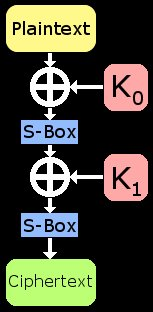# 简化目标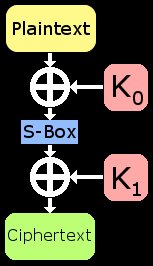# 差分性质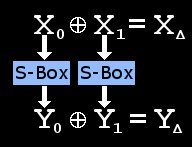# 寻找差分特征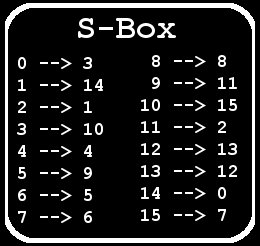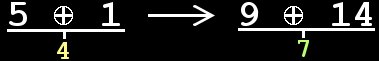# 寻找密钥